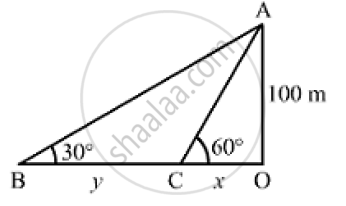# As Observed Form the Top of a Lighthouse, 100m Above Sea Level, the Angle of Depression of a Ship, Sailing Directly Towards It, Changes from 30° and 60° . - Mathematics

As observed form the top of a lighthouse, 100m above sea level, the angle of depression of a ship, sailing directly towards it, changes from 30° and 60° . Determine the distance travelled by the ship during the period of observation.

#### Solution

Let OA be the lighthouse and B and C be the positions of the ship.
Thus, we have:
OA = 100m, ∠OBA = 30° and  ∠OCA = 60°Let
OC =  xmand BC = ym
In the right ΔOAC,we have

(OA)/(OC) = tan 60° = sqrt(3)

⇒100/x = sqrt(3)

⇒ x = 100/sqrt(3) m

Now, in the right ΔOBA,we have:

(OA)/(OB) =tan 30° = 1/ sqrt(3)

⇒  100/(x+y) = 1/ sqrt(3)

⇒  x+ y = 100 sqrt(3)

On putting x = 100/ sqrt(3) in the above equation, we get:

y = 100 sqrt(3) - 100/sqrt(3) = (300-100)/ sqrt(3) = 200/sqrt(3) = 115.47 m

∴Distance travelled by the ship during the period of observation = B = y =115.47m

Concept: Heights and Distances
Is there an error in this question or solution?
Chapter 14: Height and Distance - Exercises

#### APPEARS IN

RS Aggarwal Secondary School Class 10 Maths
Chapter 14 Height and Distance
Exercises | Q 27

Share